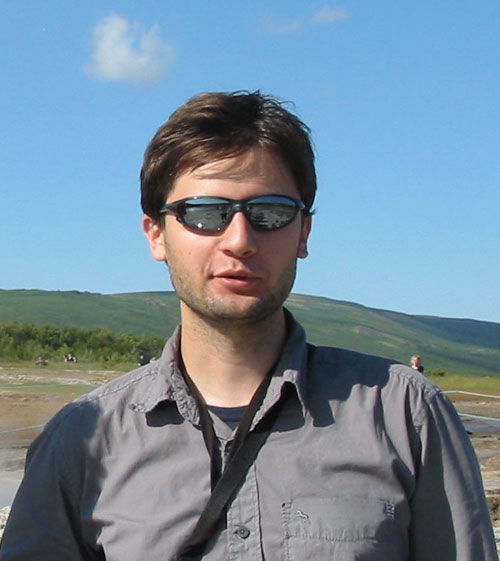# Alexandr Andoni (Theory Seminar W14)''Parallel Algorithms for Geometric Graph Problems''
Alexandr Andoni
(Microsoft Research)
Monday, February 10rd, 2014, 2:00pm
EBU3B, Room 4140
Abstract:
Motivated by modern parallel computing models (think MapReduce), we give a new algorithmic framework for geometric graph problems. Our framework applies to problems such as the Minimum Spanning Tree (MST) problem over a set of points in a low-dimensional space, which is useful for hierarchical agglomerative clustering. Our algorithm computes a (1+epsilon)-approximate MST in a *constant* number of rounds of communication, while using total space and communication proportional to the size of the data only.

Our framework proves to have implications beyond the parallel models as well. For example, we consider the Earth-Mover Distance (EMD) problem, for which we obtain a new near-linear time algorithm as well as the first streaming algorithm with subpolynomial space (assuming we can pre-sort the points). Technically, our framework for EMD shows how to effectively break up a "big Linear Programming problem" into a number of "small Linear Programming problems", which can be later recombined using a dynamic programming approach.

Joint work with Krzysztof Onak (IBM), Aleksander Nikolov (Rutgers), Grigory Yaroslavtsev (ICERM/Brown). (paper link http://arxiv.org/abs/1401.0042)